• 沈阳工程学院 源代码 ARM课程设计 课程设计 日历电子钟设计 LPC2103
• （1）通过检索、查资料和研究学习，设计应用系统原理图； （2）编写 电子日历时钟设计 应用系统程序； （3）软硬件仿真调试，实现应用系统设计目标； （4）写出设计总结报告。电子日历时钟
• 基于51单片机的LCD日历时钟毕业设计带仿真和论文.rar
• 计算机 电子信息工程 通信工程 实验 课程设计 工程项目 资源 必过 已过 好用 答辩简单 按着来就行 大学生关注我 以后所有我的课设都会更新 心得体会 参考文献自己写哈
• 该系统是以52单片机为基础，以时钟日历芯片DSl2887为核心构建的时钟系统。完成的功能如下：显示年、月、日、星期、小时、分钟、秒钟，具有1OO年的日历，具有掉电不丢失性，能够通过按键调整时间。DS12887内置锂电池...

该系统是以52单片机为基础，以时钟日历芯片DSl2887为核心构建的时钟系统。完成的功能如下：显示年、月、日、星期、小时、分钟、秒钟，具有1OO年的日历，具有掉电不丢失性，能够通过按键调整时间。DS12887内置锂电池，可以在掉电情况下工作10年。

一、芯片简介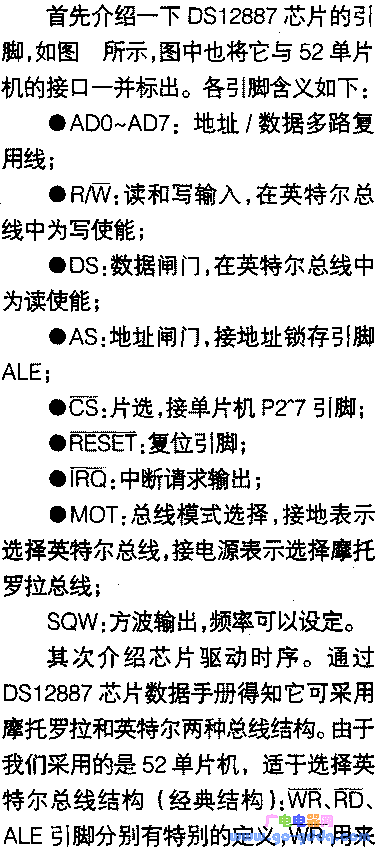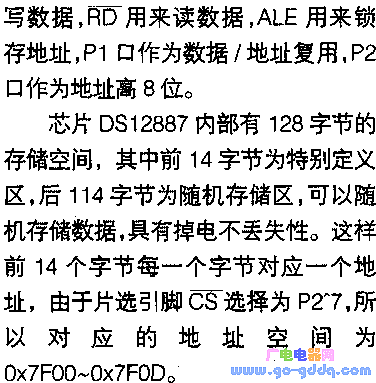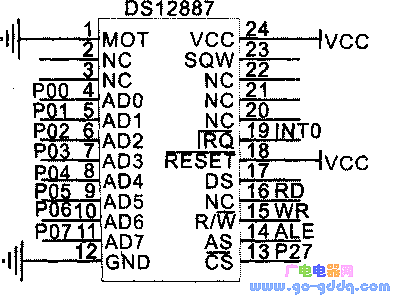在C语言程序中要读取或写入数据非常简单，只需要一条语句即可实现。在汇编语言中就是对MOVX语句的时序分析。DS12887的英特尔读周期时序如上图，写周期时序如下图所示：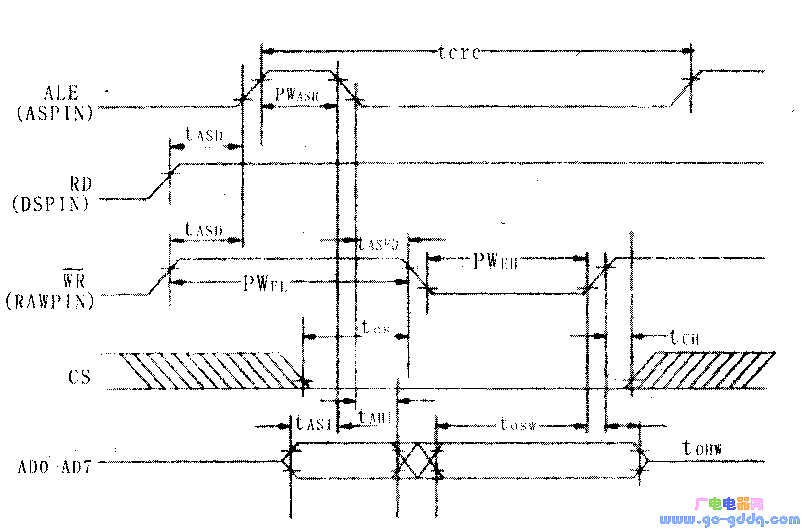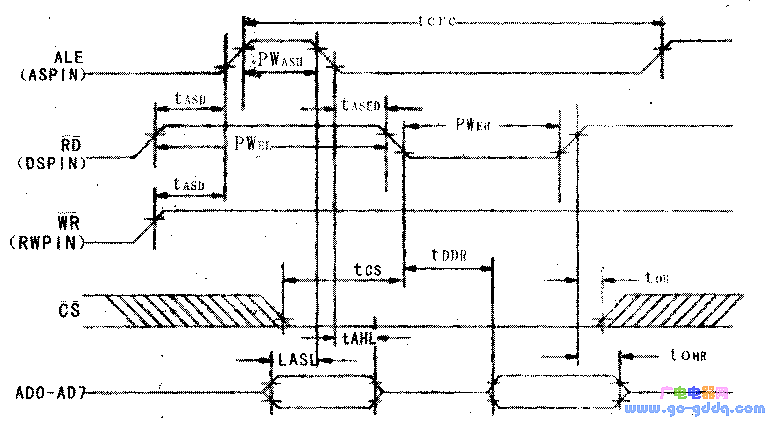最后简单介绍一下DS12887的控制字定义：

上面已经提到DSl2887内部有128字节的存储空间。其中前14字节为特别定义区(具体定义见PDF)，后114字节为随机存储区。

14字节特别定义区的具体定义如上表所示。其中0x7f00～0x7f0D是由于在本系统中选用单片机P2．7引脚接CS端所决定的。这些地址的最高位都为0，对应P2．7引脚，会使得在写入或读取的过程中在P2．7引脚上恰好产生低电平，选中芯片，完成英特尔总线时序。如果我们将单片机P2．6引脚接CS端，地址范围应为：0xbf00～0xbf0D,其中b的二进制为1011，对应会使得P2．6引脚产生低电平的片选脉冲。从0～9号地址为年月等的寄存器，用户可以读取和写入。10～13号地址为控制寄存器，寄存器D为只读，寄存器A～C的各位具体定义介绍如下(如下表)

●UlP：只读状态标志；

●DV0～2：控制芯片内部晶振位，010组合打开晶振正常工作；

●RS0～3：设定中断速率和SQW方波输出；

●SET：读写控制位，1时可以初始时钟日历字节；

●PIE、AIE、UIE：分别为周期、闹钟、更新结束中断允许位；

●PF、AF、MF：分别为周期、闹钟、更新结束中断标志位

●IRQF：中断请求标志位，IRQF=PF·PlE+AF·AlE+MF·UIE；

●SQWE：方波输出允许位；

●DM：选择数据为二进制(1)或BCD(0)码格式；

●24／12：选择时钟为24，小时格式门2小时格式；DSE：白天节时，置0就行。

地址

内容

说明

00x7f00

Seconds

0-59秒寄存器

10x7f01

SecondsAlarm

O-59秒闹钟

20x7f02

MinUteS

0-59分寄存器

3Ox7f03

MinutesAlarm

O-59分闹钟

4Ox7f04

Hours

0-23时寄存器

50x7f05

HoursAlam

O-23时闹钟

60x7f06

DayOftheWeek

1-7星期几

70x7f07

DateOftheMonth

1-31几号

80x7f08

Month

1-12月份

90x7f09

Year

0-99年数

100x7f0A

REGISTERA

控制寄存器A

110x7f0B

REGISTERB

控制寄存器B

120x7f0C

REGISTERC

控制寄存器C

130x7f0D

REGISTERD

控制寄存器D

控制寄存器

BIT7

BIT6

BIT5

BlT4

BIT3

BIT2

BIT1

BIT0

REGISTERA

UIP

DV2

DV1

DV0

RS3

RS2

RS1

RS0

REGISTERB

SET

PIE

AIE

UIE

SQWE

DM

24/12

DSE

REGISTERC

IRQF

PF

AF

MF

0

0

O

O

二、实物制作

原理图如上图所示。实际制作的电子日历系统的PCB版图如下图。在下图中WR、RD、INTO、GND和VCC标号处的焊盘是飞线用的，这里是单面板，工艺上只能达到15mil粗细程度，所以飞线之处较多。笔者认为ZLG7289在系统里边仅用来控制8个按键，有些浪费，读者可以直接挂在单片机I／O口上，不过不要忘了按键去抖，但是液晶的使用还是很有必要的，因为日历的信息比较多，如果只用8个数码管，不方便。图中考虑到在调试过程中的测试方便，将单片机的各端口都用排针引出来了。安装完成后，将程序写入，这种并口的芯片调试容易。

将图中的元器件参数列表如下：

AT89S52、DS12887、ZLG7289、LCDl602各一；C1、C2、C4、C5、C6：30pF瓷片电容；C3：1μF电解电容；C7：100μF电解电容；C8：1000μF电解电容；C9：10μF电解电容；R1、R4、R6：10kΩ欧姆电阻；R5、R7：1欧姆电阻；R2：2．2kΩ欧姆电阻；R3：1OO欧姆电阻；R11：100kΩ欧姆电阻；104、104J：100kΩ排阻；1602：16脚排针；P0，P1，P2，P3：8脚排针；SPI：6脚排针；D1：红色LED指示电源；LS1：蜂鸣器；Q1：PNP三极管9012；S1～S8：两脚封装的按键；Y1、Y2：12MHz晶振；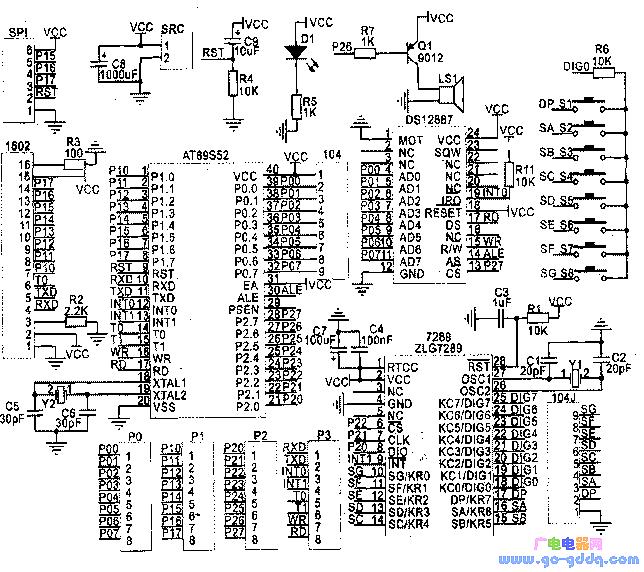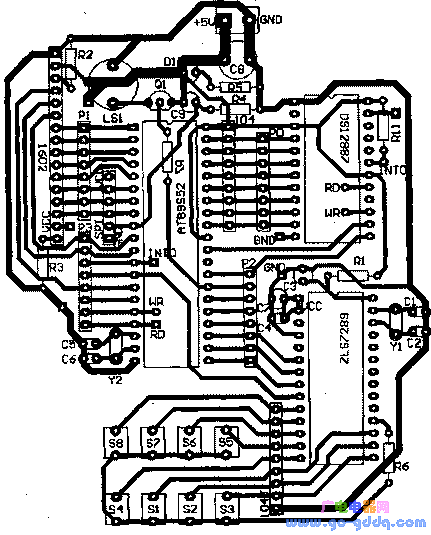三、程序设计

下面是DS12887的驱动程序和调时程序。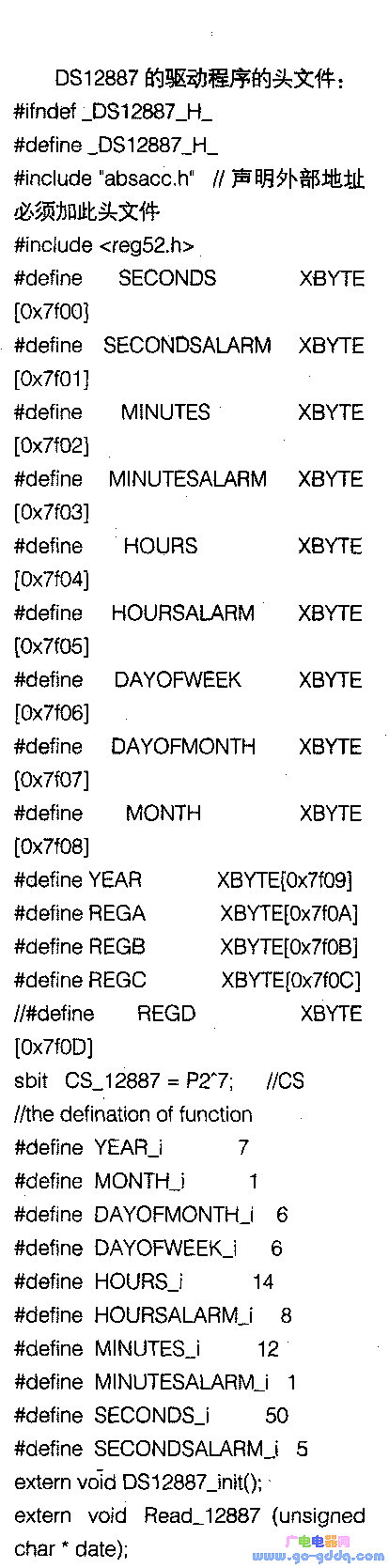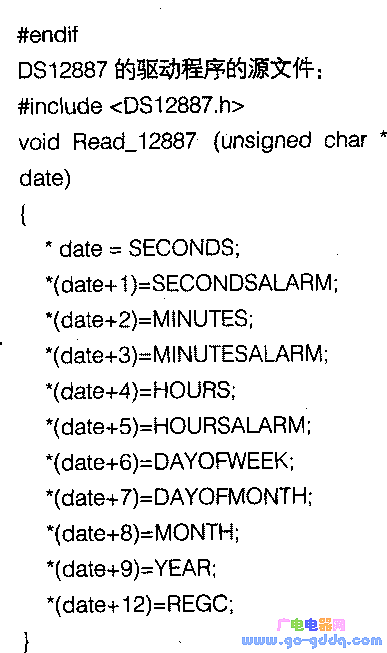我们开始要在主程序的开始设置一个初始化DS12887的函数，写入使晶振开启和设置初始时间的命令。在调试成功后，应该将这个初始化函数删掉，这样在单片机掉电又重新上电后就不用重新设置时间。

上面的程序就是删掉初始化函数后的样子。这样就完成了掉电后不会丢失时间的功能，这对于时钟日历来说，是一个非常实用的功能。

在主程序中，可以通过编程实现人机交互，完成调整DS12887内部时间的程序，还可以设定闹钟，完成报时功能。

举例如下：

开启和关闭晶振．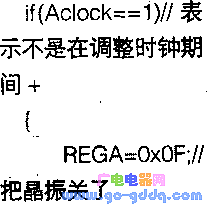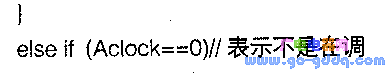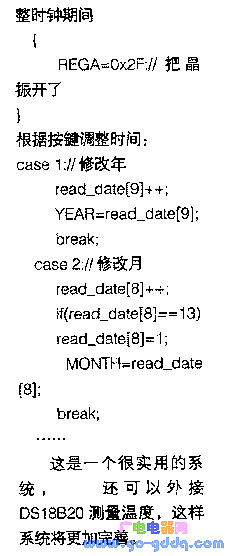展开全文• 日历电子钟设计有整点报时功能日历电子钟设计有整点报时功能日历电子钟设计有整点报时功能日历电子钟设计有整点报时功能
• 这是一个用DS1302与1602LCD设计的可调式电子日历时钟proteus仿真图
• 解决的方法有很多,这里主要阐述在Web项目中填写日历,自己动手开发一个电子日历,供用户 选择合适的日期. 基于Web的电子日历很简单,其原理如下: 构造一个日历对象 改变相应的年份和月份 显示此年此月的月历.web前端
• C51数字电子日历/时钟设计

千次阅读 多人点赞 2014-06-29 20:46:36
数字电子日历/时钟设计   设计一个基于MCS51的电子日历时钟。 基本要求 （1） 可通过按键在日历和时间之间切换显示； （2） 可由按键调整日期和时间 （3） 可整点报时（“嘟、嘟”声） （4） 可设定时，...

题目要求： 数字电子日历/时钟设计

设计一个基于MCS51的电子日历和时钟。

基本要求

（1） 可通过按键在日历和时间之间切换显示；

（2） 可由按键调整日期和时间

（3） 可整点报时（“嘟、嘟”声）

（4） 可设定时，定时时间到发出“嘟、嘟”声

（5） 具有秒表功能

课程设计电路图：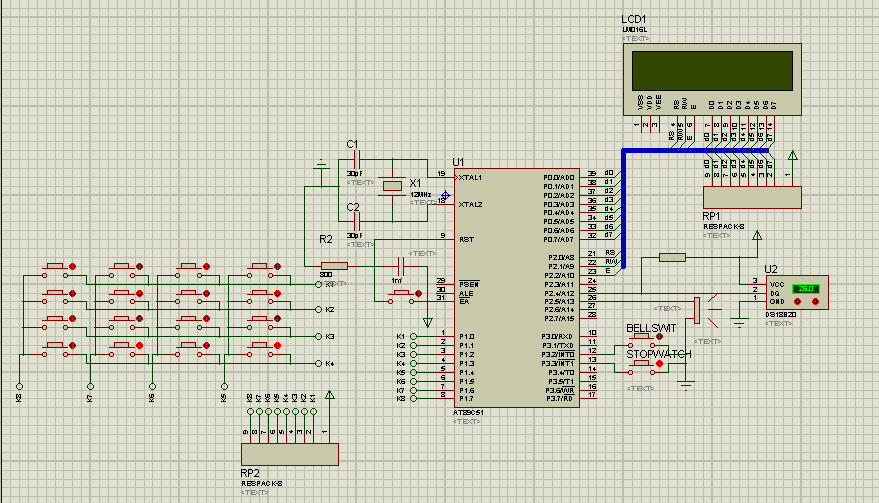该课程设计采用ATMEL公司的FLASH 型经典芯片——AT89C51系列单片机作为时钟的控制核心，用于自动显示当前时间、日期、星期、温度。

利用单片机定时器T0的中断程序设计出一秒钟的精确定时，通过单一按钮实现了当前时间、日期、秒表、设定闹钟、温度测量等功能的切换，通过矩阵键盘编程实现设置年、月、日、时、分、秒的功能，也可以设定闹钟时间。闹钟可以自定义开关，并具有贪睡功能，闹钟到达预设的时间时，利用扬声器发出“嘟、嘟”声音。系统具有实现整点报时功能。最后在设计中时附加了测温功能，利用DS18B20实时测试环境的温度。

该设计实现功能：

（1） 可通过按键在日历和时间之间切换显示；

（2） 可由按键调整日期和时间；

（3） 可整点报时（“嘟、嘟”声）；

（4） 可设定时，定时时间到发出“嘟、嘟”声，并具有贪睡功能和开关功能；

（5） 具有秒表功能；

（6） 具有实时温度测量功能；

（7） 具有星期查询功能；

由于C语言程序设计较汇编可读性强，可移植性，且可以大大降低编程的难度和缩短开发周期，本系统程序采用C语言设计。

软件设计思想：

（1）单片机控制模块：单片机控制模块在系统中处于核心地位，其工作包括读取并处理键盘输入、显示模块控制、显示模块切换、闹钟控制等任务。

（2）按键输入模块：此模块完成对各种功能的控制，功能的切换在硬件上通过此部分来操作完成。

（3）温度传感器模块：此模块完成测温功能，通过温度传度器对外部温度的读取，并将信号输入单片机，单片机将此信号进行处理，并在LCD1602上显示。

（4）闹钟模块：此模块实现时钟的整点报时，定时响铃。系统正常工作后，每到整点或闹钟设定时间时，扬声器会发出“嘟、嘟”声。

使用定时器T0定时50ms用于时钟计时，定时器T1定时10ms用于秒表计时，外部中断0用于设定定时开关，外部中断1用于设定秒表的计时和清零。

初值计算：单片机主频12MHz,机器周期为1微妙，定时器T0使用方式1定时50ms，则计数初值为216-(50ms/1us)=65536-50000=0x3cb0;定时器T1使用方式1定时10ms，则计数初值为216-(10ms/1us)=65536-10000=0xd8f0。

源代码：

/*Main.c*/

#include<reg51.h>

#include"LCD1602.h"

#include"KeyScan.h"

#include"SetValue.h"

#include"Sounder.h"

#include"Ds18b20.h"

intIntCount=0,StopCount=0,StopMin=0;

ucharSec=0,Min=0,Hour=0,Date=1,month=1,SetMin=1,SetHour=0,NUM1,CountWeek;

intyear=2014,Qiehuan;

char KEY;

unsignedchar code dis_week[]={"SUN,MON,TUE,WED,THU,FRI,SAT"};

unsignedchar code para_month={0,0,3,3,6,1,4,6,2,5,0,3,5};      //星期月参变数

ucharcode Timetable1[]="  CurrentTime  ";

ucharcode Datetable1[]="  CurrentDate  ";

ucharcode Settable1[]=" Set RingTime ";

ucharcode CurrentTime[]="   00:00:00    ";

ucharcode SetTime[]="     00:00      ";

ucharcode CurrentDate[]="  2014-01-01   ";

ucharcode CurrentTemp[]=" Temperature  ";

ucharcode Temptable[]="     00.0'C     ";

ucharcode Stopwatch[]="    StopWatch   ";

ucharcode Stoptable[]="    000.00s     ";

bitalarm;

/*定时器0、1初始化*/

voidTimer_Init(void)

{

TMOD=0x11;                 /*定时器T0、T1初始化为方式1*/

TL0=0xb0;                                 /*装入定时初值，在主频12MHZ下，定时50ms*/

TH0=0x3c;

TL1=0xf0;                                  /*装入定时初值，在主频12MHZ下，定时10ms*/

TH1=0xd8;

ET0=1;                      /*开启定时器T0中断*/

ET1=1;                      /*开启定时器T1中断*/

TR0=1;                                      /*启动定时器T0定时*/

TR1=0;

}

/*外部中断0初始化*/

voidInt_Init(void)

{

IT0=1;    //设置下降沿触发方式

EX0=1;     //开放外部中断0

IT1=1;    //设置下降沿触发方式

EX1=1;     //开放外部中断0

EA=1;

}

/*外部中断0中断函数*/

voidInt0_int(void) interrupt 0

{

alarm=!alarm;          //闹钟的开关切换

}

/*中断号1是定时器T0中断*/

voidTimer0_int(void) interrupt 1

{

EA=0;                                        //关中断

TL0=0xb7;                                 //重装定时初值，在主频12MHZ下，定时50ms

TH0=0x3c;                                 //修正量为7个机器周期

IntCount++;

if(IntCount==20)

{

Sec++;                    //秒位加一

IntCount=0;

}

EA=1;                                 //开中断

}

/*外部中断1的中断函数，设定秒表的起始*/

voidInt1_int(void) interrupt 2

{

TR1=!TR1;

if(TR1)

StopMin=0;

}

/*中断号3是定时器T1中断*/

void Timer1_int(void) interrupt 3

{

EA=0;                          /*关中断*/

TL1=0xf0;                    /*装入定时初值，在主频12MHZ下，定时10ms*/

TH1=0xd8;

StopMin++;

EA=1;

}

/*按键处理程序*/

voidKey_Process(uchar Qkeynum)

{

if(Qkeynum=='1')  //按键一用于显示切换

{

Qiehuan++;

if(Qiehuan==1)          //显示当前时间

{

uchar num;

write_com(0x01);  //显示清屏

write_com(0x80);

for(num=0;num<15;num++)

{

write_data(Timetable1[num]);

delay(5);

}

write_com(0x80+0x40);

for(num=0;num<16;num++)

{

write_data(CurrentTime[num]);

delay(5);

}

}

else if(Qiehuan==2)       //显示当前日期

{

uchar num;

write_com(0x01);//显示清屏

write_com(0x80);

for(num=0;num<15;num++)

{

write_data(Datetable1[num]);

delay(5);

}

write_com(0x80+0x40);

for(num=0;num<16;num++)

{

write_data(CurrentDate[num]);

delay(5);

}

}

else if(Qiehuan==3)              //显示当前定时

{

uchar num;

write_com(0x01);//显示清屏

write_com(0x80);

for(num=0;num<15;num++)

{

write_data(Settable1[num]);

delay(5);

}

write_com(0x80+0x40);

for(num=0;num<16;num++)

{

write_data(SetTime[num]);

delay(5);

}

}

else if(Qiehuan==4)              //显示当前温度

{

uchar num;

write_com(0x01);//显示清屏

write_com(0x80);

for(num=0;num<15;num++)

{

write_data(CurrentTemp[num]);

delay(5);

}

write_com(0x80+0x40);

for(num=0;num<16;num++)

{

write_data(Temptable[num]);

delay(5);

}

}

else if(Qiehuan==5)        //显示秒表

{

uchar num;

write_com(0x01);//显示清屏

write_com(0x80);

for(num=0;num<15;num++)

{

write_data(Stopwatch[num]);

delay(5);

}

write_com(0x80+0x40);

for(num=0;num<16;num++)

{

write_data(Stoptable[num]);

delay(5);

}

Qiehuan=0;

}

}

elseif(Qkeynum=='2')

{

Sec++;

if(Sec>59)

Sec=0;

}

if(Qkeynum=='3')

{

Min++;

if(Min>59)

Min=0;

}

if(Qkeynum=='4')

{

Hour++;

{

if(Hour>23)

Hour=0;

}

}

if(Qkeynum=='5')

{

Date++;

if(month==1||month==3||month==5||month==7||month==8||month==10||month==12)

if (Date>31)

{

Date=1;

}                                 //大月31天

else if(month==4||month==6||month==9||month==11)

if (Date>30) {Date=1;}                               //小月30天

}

if(Qkeynum=='6')

{

month++;

if (month>12)

month=1;

}

if(Qkeynum=='7')

{

year++;

if(year>2099)

year=2000;

}

if(Qkeynum=='8')

{

SetMin++;

if(SetMin>59)

SetMin=0;

}

if(Qkeynum=='9')

{

SetHour++;

if(SetHour>23)

SetHour=0;

}

if(Qkeynum=='A') //设置贪睡功能

{

SetMin=SetMin+2;

if(SetMin>60)

{

SetMin=SetMin-60;

SetHour++;

if(SetHour>23)

SetHour=0;

}

}

}

/*闰年的计算*/

bitleap_year()

{

bit leap;

if((year%4==0&&year%100!=0)||year%400==0)//闰年的条件

leap=1;

else

leap=0;

return leap;

}

/*星期的自动运算和处理*/

/*基姆拉尔森计算公式*/

unsignedchar week_proc()

{     unsigned char num_leap;

unsigned char c;

unsigned char week;

num_leap=year/4-year/100+year/400;//自00年起到year所经历的闰年数

if( leap_year()&& month<=2 )       //既是闰年且是1月和2月

c=5;

else

c=6;

week=(year+para_month[month]+Date+num_leap+c+4)%7;//计算对应的星期

return week;

}

/*显示当前时间*/

voidShowTime(void)

{

write_com(0x80+0x40);

write_Dec(4,Hour);

write_Dec(7,Min);

write_Dec(10,Sec);

}

/*显示闹钟设定时间*/

voidShowSetTime(void)

{

write_com(0x80+0x40);

write_Dec(5,SetHour);

write_Dec(8,SetMin);

}

/*显示日期*/

voidShowDate(void)

{

write_com(0x80+0x40);

write_Dec(2,20);

write_Dec(4,(year%100));

write_Dec(7,month);

write_Dec(10,Date);

}

/*显示秒表时间*/

voidShowStopWatch()

{

write_com(0x80+0x40+4);

write_data(StopMin/10000+0x30);

write_data((StopMin%10000)/1000+0x30);

write_data((StopMin%1000)/100+0x30);

write_com(0x80+0x40+8);

write_data((StopMin%100)/10+0x30);

write_data((StopMin%10)+0x30);

}

voidCalcu_time(void)

{

if(Sec>59)

{

Sec=0;

Min++;

if(Min>59)

{

Min=0;

Hour++;

if(Hour>23)  //*24H从零点开始

{

Date++;

Hour=0;

if(month==1||month==3||month==5||month==7||month==8||month==10||month==12)

if (Date>31)

{Date=1;month++;

}                              //大月31天

else if(month==4||month==6||month==9||month==11)

if (Date>30) {Date=1;month++;}                                 //小月30天

else if (month==2)

{

if(leap_year())                                                   //闰年的条件

{

if (Date>29)

{

Date=1;month++;

}

}                     //闰年2月为29天

else

{

if (Date>28) {Date=1;month++;}            //平年2月为28天

}

}    //if (month==2)

else if (month>12)

{

month=1;

year++;

if(year>2099)

year=2000;

}   //if (month>12)

}     //if(Hour==24)

}           //if(Min==60)

}  //if(Sec==60)

if(Qiehuan==2)        //日期和星期同时显示

{

CountWeek=week_proc();

write_com(0x80+0x40+13);

write_data(dis_week[4*CountWeek]);

write_data(dis_week[4*CountWeek+1]);

write_data(dis_week[4*CountWeek+2]);

}

if((Min==SetMin)&&(Hour==SetHour))     //闹钟定时到达后响铃

{

if (alarm)

BellRing();

else Sounder=0;

}

else Sounder=0;           //扬声器关闭

if (alarm&&(Qiehuan==1))     //闹钟开关标志和时间同时显示

{

write_com(0x80+0x40);

write_data('O');

write_data('N');

}

else if((!alarm)&&(Qiehuan==1))

{

write_com(0x80+0x40);

write_data('O');

write_data('F');

}

}

void main()

{

LCD_init();       //LCD1602初始化

Timer_Init();  //定时器初始化

Int_Init();          //外部中断初始化

Qiehuan=1;

while(1)

{

Calcu_time();         //时间累计及显示

if(Qiehuan==1)

{

ShowTime();  //在LCD1602上显示时间

}

else if(Qiehuan==2)

{

ShowDate();

}

else if(Qiehuan==3)

{

ShowSetTime();

}

elseif(Qiehuan==4)

{

ds18b20Process();  //显示温度

}

else if (Qiehuan==0)

{

ShowStopWatch();   //显示秒表

}

WholePoint();   //整点报时函数

KEY=keynum();        //按键检测及扫描

if(KEY!=0)

{

NUM1=coding(KEY);

Key_Process(NUM1);

}

}

}

/*LCD1602.c*/

#include"LCD1602.h"

#include"SetValue.h"

ucharcode Timetable[]="  CurrentTime  ";

ucharcode Datetable[]="  CurrentDate  ";

ucharcode Currenttable[]="   00:00:00    ";

voidLCD_init(void)

{

uchar num;

rw=0;

write_com(0x38);//显示模式设置

write_com(0x0c); //开显示，不显示光标

write_com(0x06); //地址指针加一，光标加一

write_com(0x01);//显示清屏

write_com(0x80);

for(num=0;num<15;num++)

{

write_data(Timetable[num]);

delay(5);

}

write_com(0x80+0x40);

for(num=0;num<16;num++)

{

write_data(Currenttable[num]);

delay(5);

}

}

voiddelay(uint z)

{

uint x,y;

for(x=z;x>0;x--)

for(y=110;y>0;y--);

}

/*写指令：RS=L,RW=L,D0~D7=指令码，E=高脉冲*/

voidwrite_com(unsigned char com)

{

rs=0;

lcden=0;

P0=com;

delay(5);

lcden=1;

delay(5);

lcden=0;

}

/*写数据：RS=H,RW=L,D0~D7=指令码，E=高脉冲*/

voidwrite_data(unsigned char Data)

{

rs=1;

lcden=0;

P0=Data;

delay(5);

lcden=1;

delay(5);

lcden=0;

}

{

unit=date%10;

write_data(0x30+unit);

}

/*KeyScan.c*/

#include<KeyScan.h>

#include<reg51.h>

#include"SetValue.h"

#include"LCD1602.h"

unsigned char code a[]={0xFE,0xFD,0xFB,0xF7};

/*获得按键对应的键值*/

unsigned char coding(unsigned char m)

{

unsigned char k;

switch(m)

{

case (0x18):k='1';break;

case (0x28):k='2';break;

case (0x48):k='3';break;

case (0x88):k='4';break;

case (0x14):k='5';break;

case (0x24):k='6';break;

case (0x44):k='7';break;

case (0x84):k='8';break;

case (0x12):k='9';break;

case (0x22):k='A';break;

case (0x42):k='9';break;

case (0x82):k='C';break;

case (0x11):k='*';break;

case (0x21):k='0';break;

case (0x41):k='#';break;

case (0x81):k='D';break;

}

return(k);

}

//=====================按键检测并返回按键值==========================

unsigned char keynum(void)

{

unsigned char row,col,i;

P1=0xf0;   //低四位输出低电平

if((P1&0xf0)!=0xf0)   //P1口输入值是否为0xf0，不是则有键按下

{

delay(10);        //延时按键消抖

if((P1&0xf0)!=0xf0)

{

row=P1^0xf0;          //异或确定哪列有键按下

i=0;

P1=a[i];             //精确定位某列的按键

while(i<4)

{

if((P1&0xf0)!=0xf0)

{

col=~(P1&0xff);   //确定行线

break;            //已定位后提前退出

}

else

{

i++;

P1=a[i];

}

}

}

else

{

return 0;

}

while((P1&0xf0)!=0xf0);   //等待按键释放

return (row|col);          //行线与列线组合后返回

}

else return 0;                  //无键按下时返回0

}

/*Sounder.c*/

#include"SetValue.h"

void Soundelay(uint z)

{

uint x,y;

for(x=z;x>0;x--)

for(y=110;y>0;y--);

}

/*引脚取反产生高低电平使扬声器发声*/

void BellRing(void)

{

Sounder=!Sounder;

Soundelay(50);

}

/*整点报时函数*/

void WholePoint(void)

{

if((Min==0)&&(Sec<3))

BellRing();

else Sounder=0;

}

/*ds18b20.c*/

#include<reg51.h>

#include"LCD1602.h"

sbit DQ        = P2 ^4;  //ds1820data

void DSDelay(int num)//延时函数

{

while(num--) ;

}

/*初始化ds18b20,参见datasheet初始化时序图*/

void Init_DS18B20(void)//

{

unsigned char x=0;

DQ = 1;    //DQ复位

DSDelay(8);  //稍做延时

DQ = 0;    //单片机将DQ拉低

DSDelay(80); //精确延时 大于 480us

DQ = 1;    //拉高总线

DSDelay(14);

x=DQ; //稍做延时后ds18b20发出存在脉冲，若x=0则初始化成功 x=1则初始化失败

DSDelay(20);

}

/**********************************************************************/

{

unsigned char i=0;

unsigned char dat = 0;

for (i=8;i>0;i--)

{

/*当总线控制器把数据线从高电平拉到低电平，读时序开始*/

DQ = 0; // 给脉冲信号

dat>>=1;

DQ = 1; // 给脉冲信号

if(DQ)//ds18b20通过拉高或拉低总线来传递1或0

dat|=0x80;

DSDelay(4);

}

return(dat);

}

/***********************************************************/

void WriteOneChar(unsigned char dat)//写一个字节

{

unsigned char i=0;

for (i=8; i>0; i--)

{

DQ = 0;   //数据线拉低再释放，写时序开始

DQ = dat&0x01;  //写0时序或写1时序

DSDelay(5);

DQ = 1;

dat>>=1;

}

}

/*读取温度*/

{

unsigned char a=0;

unsigned char b=0;

unsigned int t=0;

float tt=0;

Init_DS18B20();

WriteOneChar(0xCC); //跳过读序号列号的操作

WriteOneChar(0x44); //启动温度转换

Init_DS18B20();

WriteOneChar(0xCC); //跳过读序号列号的操作

WriteOneChar(0xBE); //读取温度寄存器

t=b;

t<<=8;

t=t|a;

tt=t*0.0625;//0.0625/LSB

t= tt*10+0.5; //放大10倍输出并四舍五入

return(t);

}

void ds18b20Process(void)

{

unsigned int i=0;

unsigned chara=0,b=0,c=0,f=0;

a=i/100;

b=i/10-a*10;

c=i-a*100-b*10;

write_com(0x80+0x40+5);

write_data(0x30+a);

write_data(0x30+b);

write_com(0x80+0x40+8);

write_data(0x30+c);

}

/*SetValue.h*/

#ifndef _SetValue_H_

#define _SetValue_H_

#include<reg51.h>

#define uchar unsigned char

#define uint unsigned int

sbit rs=P2^0;

sbit rw=P2^1;

sbit lcden=P2^2;

sbit Sounder=P2^5;

extern uchar SetHour;

extern uchar SetMin;

extern int IntCount;

extern uchar Sec;

extern uchar Min;

extern uchar Hour;

extern uchar Date;

extern uchar month;

extern int year;

#endif

/*1602.h*/

#ifndef _LCD1602_H_

#define _LCD1602_H_

void LCD_init(void);

void delay(unsigned int z);

void write_com(unsigned char com);

void write_data(unsigned char Data);

void write_Dec(unsigned char add,unsigned char date);

#endif

/*Sounder.h*/

void BellRing(void);

void WholePoint(void);

/*KeyScan.h*/

#ifndef _KeyScan_H_

#define _KeyScan_H_

unsigned char coding(unsigned char m);

unsigned char keynum(void);

#endif

展开全文单片机 设计 proteus
• 计算机 电子信息工程 通信工程 实验 课程设计 工程项目 资源 必过 已过 好用 答辩简单 按着来就行 大学生关注我 以后所有我的课设都会更新
• 介绍一种显示时间、日期和星期的电子日历钟, 对星期的日进位信号提出三种设计方案以及按键输入电路的设计方法.电子日历
• 一、 任务和要求设计制作一个电子表并且能够显示日历，用LED数码管直接显示，并且能够通过按键调整时间、年月日，功能为按第一个键被调整的相应调整区域开始闪烁，第二个键加一，第三个减一，第四个确认，最后一个在...

一、 任务和要求

设计制作一个电子表并且能够显示日历，用LED数码管直接显示，并且能够通过按键调整时间、年月日，功能为按第一个键被调整的相应调整区域开始闪烁，第二个键加一，第三个减一，第四个确认，最后一个在时分秒和年月日中间相互切换。

二、方案论证

我们在这里选用了C8051F单片机，它是完全集成的混合信号系统级芯片(SoC)，具有与8051兼容的高速CIP-51内核，与MCS-51指令集完全兼容，片内集成了数据采集和控制系统中常用的模拟、数字外设及其他功能部件；内置FLASH程序存储器、内部RAM，大部分器件内部还有位于外部数据存储器空间的RAM，即XRAM。C8051F单片机具有片内调试电路，通过4脚的JTAG接口可以进行非侵入式、全速的在系统调试。本文引用地址：http://www.eepw.com.cn/article/201611/321849.htm

三、 系统硬件电路设计

该系统分为两个部分，主控电路和扫描电路，其中键盘扫描和LED扫描为今后节省I/O口线，我将键盘的扫描和LED的扫描共用，在扫描LED的同时也扫描键盘，这样不但方便，而且为今后的扩展留下了相当大的余地。

3.1主控制器

单片机使用c8051f310，并且使用它的开发板易于烧录，改变程序和外围电路。

3.2显示电路

显示板因为常用，是我自己画出来交给工厂做的，采用4个两位共阳数码管，从P0口输出，8位段码分别和P0相接，通过定时器实现秒钟的加一，又因为310有推挽输出，所以不必再加外部电路对数码管进行驱动，直接将它们与P0口相连就可以

3.3键盘电路

键盘是是与数码管的位选相连，通过二极管使它们具有单向性，同时将开头最终通过三极管接单片机的P3.4，只要有按键按下，P3.4就一定会发生变化，通过这个变化在去扫描位就可以得出是哪个按键了。

附电路图：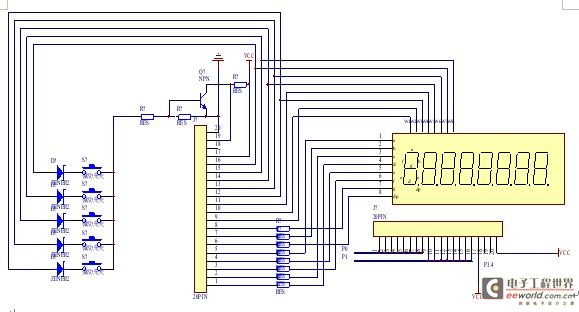四、系统程序设计

系统和程序主要包括：显示程序，键盘扫描程序，自动加秒，分，时，日，月，年，

附程序：

#include "c8051f310.h"

#define uchar unsigned char

#define unchar unsigned char

//段码表 0 1 2 3 4 5 6 7 8 9

uchar code wei={0x02,0x01,0x08,0x04,0x20,0x10,0x80,0x40};//位码表

uchar dataa,sec,min,hour,ymd,year,year1=9,year2=20,month=8，day=22,week=6;//初始值是7-00:00:00,日期是2009-08-22；

uchar n,cnt,sw1=0,sw2,sw3,cnt1,sw5;

uchar key1,key2,key3,key4=0,key5=0;

sbit P3_4=P3^4;

void scan_key(void);

void delay(ms);

void init(void)

{

IE =0x8A;

TCON =0x50;

TMOD =0x11;

IP =0x0A;

TH0 =(65536-1270)/256;

TL0 =(65536-1270)%256;

TH1 =(65536-500)/256;

TL1 =(65536-500)%256;

}

void time0(void) interrupt 1

{

TH0 =(65536-1270)/256;

TL0 =(65536-1270)%256;

dataa++;

if(sw1!=0&&key4==0) //第一个按键按下，且第四个没有按下

{

if(++cnt1==40)

{

cnt=0;

if(sw5==0&&sw1==1)

{

for(n=0;n<20;n++)

{

P1 =0x00;

P1 =P1&0xf3; //闪烁程序

P0 =duan[sec%10];

P1 =wei;

delay(8);

P1 =0x00;

P0 =duan[sec/10];

P1 =wei;

delay(8);

P1 =0x00;

P0 =duan[hour%10];

P1 =wei;

delay(8);

P1 =0x00;

P0 =duan[hour/10];

P1 =wei;

delay(8);

P1=0x00;

P0 =0x7f;

P1 =wei;

delay(8);

P1=0x00;

P0 =week1[week];

P1 =wei;

delay(8);

}

scan_key();

}

if(sw5==0&&sw1==2)

{

for(n=0;n<20;n++)

{

P1 =0x00;

P1 =P1&0xcf;

P0 =duan[sec%10];

P1 =wei;

delay(8);

P1 =0x00;

P0 =duan[sec/10];

P1 =wei;

delay(8);

P1 =0x00;

P0 =duan[min%10];

P1 =wei;

delay(8);

P1 =0x00;

P0 =duan[min/10];

P1 =wei;

delay(8);

P1 =0x00;

P0 =0x7f;

P1 =wei;

delay(8);

P1 =0x00;

P0 =week1[week];

P1 =wei;

delay(8);

}

scan_key();

}

if(sw5==0&&sw1==3)

{

for(n=0;n<20;n++)

{

P1 =0x00;

P1 =P1&0x7f;

P0 =duan[sec%10];

P1 =wei;

delay(8);

P1 =0x00;

P0 =duan[sec/10];

P1 =wei;

delay(8);

P1 =0x00;

P0 =duan[min%10];

P1 =wei;

delay(8);

P1 =0x00;

P0 =duan[min/10];

P1 =wei;

delay(8);

P1=0x00;

P0=duan[hour%10];

P1=wei;

delay(8);

P1 =0x00;

P0 =duan[hour/10];

P1 =wei;

delay(8);

P1 =0x00;

P0 =0x7f;

P1 =wei;

delay(8);

}

scan_key();

}

if(sw5==1&&sw1==9)

{

for(n=0;n<20;n++)

{

P1 =0x00;

P1 =P1&0x3f;

P0 =duan[month%10];

P1 =wei;

delay(8);

P1 =0x00;

P0 =duan[month/10];

P1 =wei;

delay(8);

P1 =0x00;

P0 =duan[year1%10];

P1 =wei;

delay(8);

P1 =0x00;

P0 =duan[year1/10];

P1 =wei;

delay(8);

P1 =0x00;

P0 =duan[year2%10];

P1 =wei;

delay(8);

P1 =0x00;

P0 =duan[year2/10];

P1 =wei;

delay(8);

}

scan_key();

}

if(sw5==1&&sw1==8)

{

for(n=0;n<20;n++)

{

P1 =0x00;

P1 =P1&0xf3;

P0 =duan[day%10];

P1 =wei;

delay(8);

P1 =0x00;

P0 =duan[day/10];

P1 =wei;

delay(8);

P1 =0x00;

P0 =duan[year1%10];

P1 =wei;

delay(8);

P1 =0x00;

P0 =duan[year1/10];

P1 =wei;

delay(8);

P1 =0x00;

P0 =duan[year2%10];

P1 =wei;

delay(8);

P1 =0x00;

P0 =duan[year2/10];

P1 =wei;

delay(8);

}

scan_key();

}

if(sw5==1&&sw1==7)

{

for(n=0;n<20;n++)

{

P1 =0x00;

P1 =P1&0xc3;

P0 =duan[day%10];

P1 =wei;

delay(8);

P1 =0x00;

P0 =duan[day/10];

P1 =wei;

delay(8);

P1 =0x00;

P0 =duan[month%10];

P1 =wei;

delay(8);

P1 =0x00;

P0 =duan[month/10];

P1 =wei;

delay(8);

P1 =0x00;

P0 =duan[year2%10];

P1 =wei;

delay(8);

P1 =0x00;

P0 =duan[year2/10];

P1 =wei;

delay(8);

}

scan_key();

}

if(sw5==1&&sw1==6)

{

for(n=0;n<20;n++)

{

P1 =0x00;

P1 =P1&0xc3;

P0 =duan[day%10];

P1 =wei;

delay(8);

P1 =0x00;

P0 =duan[day/10];

P1 =wei;

delay(8);

P1 =0x00;

P0 =duan[month%10];

P1 =wei;

delay(8);

P1 =0x00;

P0 =duan[month/10];

P1 =wei;

delay(8);

P1 =0x00;

P0 =duan[year1%10];

P1 =wei;

delay(8);

P1 =0x00;

P0 =duan[year1/10];

P1 =wei;

delay(8);

}

scan_key();

}

}

}

}

void time1(void) interrupt 3

{

TH1 =(65536-500)/256;

TL1 =(65536-500)%256;

if(++cnt>8) cnt=0;

if(sw5==0) //显示时分秒

{switch(cnt)

{

case 0: P1 =0x00;

P0 =duan[sec%10];

P1 =wei[cnt]; break;

case 1: P1 =0x00;

P0 =duan[sec/10];

P1 =wei[cnt];break;

case 2: P1 =0x00;

P0 =duan[min%10];

P1 =wei[cnt]; break;

case 3: P1 =0x00;

P0 =duan[min/10];

P1 =wei[cnt];

scan_key(); break;

case 4: P1 =0x00;

P0 =duan[hour%10];

P1 =wei[cnt];

scan_key();break;

case 5: P1 =0x00;

P0 =duan[hour/10];

P1 =wei[cnt];

scan_key();break;

case 6: P1 =0x00;

P0 =0x7f;

P1 =wei[cnt];

scan_key(); break;

case 7: P1 =0x00;

P0 =week1[week];

P1 =wei[cnt];

scan_key();break;

default: break;

}

}

if(sw5==1) //显示年月日

{switch(cnt)

{

case 0: P1 =0x00;

P0 =duan[day%10];

P1 =wei[cnt]; break;

case 1: P1 =0x00;

P0 =duan[day/10];

P1 =wei[cnt];break;

case 2: P1 =0x00;

P0 =duan[month%10];

P1 =wei[cnt]; break;

case 3: P1 =0x00;

P0 =duan[month/10];

P1 =wei[cnt];

scan_key(); break;

case 4: P1 =0x00;

P0 =duan[year1%10];

P1 =wei[cnt];

scan_key();break;

case 5: P1 =0x00;

P0 =duan[year1/10];

P1 =wei[cnt];

scan_key();break;

case 6: P1 =0x00;

P0 =duan[year2%10];

P1 =wei[cnt];

scan_key(); break;

case 7: P1 =0x00;

P0 =duan[year2/10];

P1 =wei[cnt];

scan_key();break;

default: break;

}

}

}

void conv(void)

{

if(dataa>=201)

{dataa=0;sec++;}

if(sec>=60)

{sec=0;min++;}

if(sw1==0&&min>=60)

{min=0;hour++;}

if(sw1==0&&hour>=24)

{

if(year%4==0)

{

if(month==2)

{

if(day==29)

{

day =1;

month++;

}

else day++;

}

else

{

if(month==4||month==6||month==9||month==11)

{

if(day==30)

{

day =1;

month++;

}

else day++;

}

else

{

if(day==31)

{

day =1;

month++;

if(month==13)

{

month =1;

year++;

}

}

else day++;

}

}

}

展开全文• 51单片机时钟万年历、lcd1602显示，ds1302时钟模块，可按键设置时间，可设置闹钟，keil工程加proteus,protel dxp 2004原理图,PCB图纸，本人精心设计，已做好实物，实测好用。
• 题10 数字电子日历时钟设计 设计一个基于MS尸430的电子日历时钟。 基本要求 (1）可通过按键在日历和时间之间切换显示； (2）可由按键调整日期和时间 (3）可整点报时（‘铆、嘟”声） (4）可设定时，定时时间到发出...

题10 数字电子日历/时钟设计
设计一个基于MSP430的电子日历和时钟。
基本要求
(1）可通过按键在日历和时间之间切换显示；
(2）可由按键调整日期和时间
(3）可整点报时（‘铆、嘟”声）
(4）可设定时，定时时间到发出嘟、嘟”声
(5）具有秒表功能
设计以MSP430为核心的控制电路并编写相关的系统软件。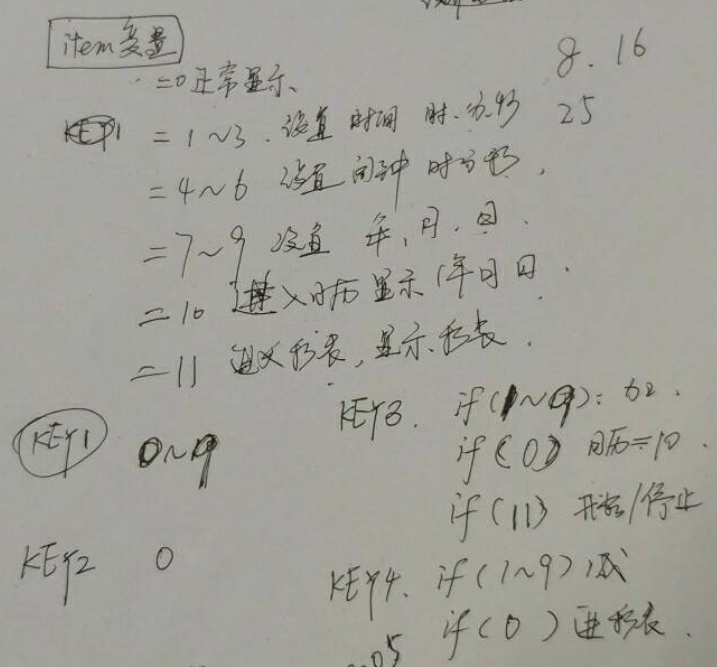时钟走时：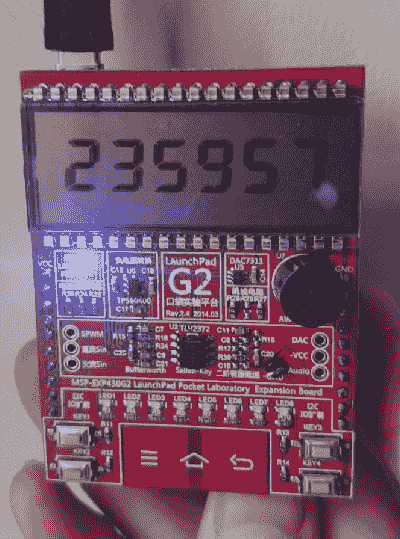秒表：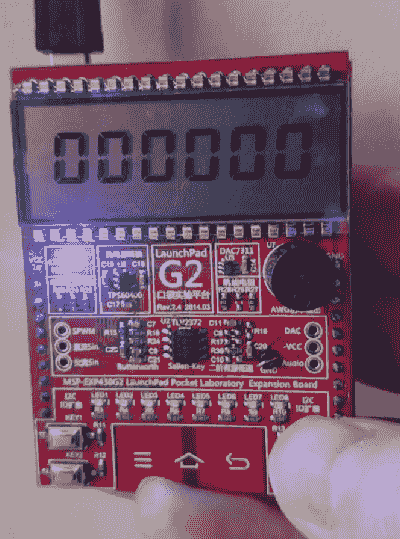日期切换：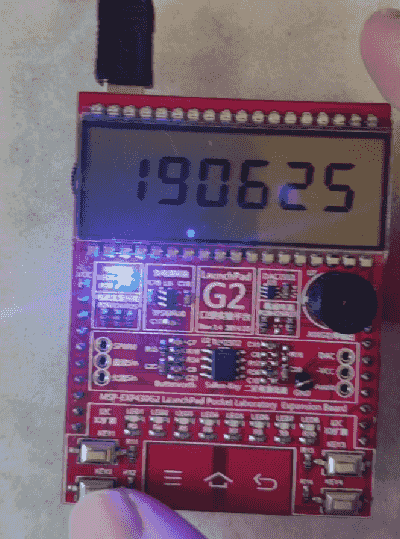展开全文电子时钟 万年历
• 计算机 电子信息工程 通信工程 实验 课程设计 工程项目 资源 必过 已过 好用 答辩简单 按着来就行 大学生关注我 以后所有我的课设都会更新 所需积分很低 签一次到就能得不用去桃宝买 多支持 个人主页有更多课设实验...
• 用DS1302与1602LCD设计的可调式电子日历时钟设计与实现程序,很有用的.DS1302
• 可以查询日历，可以设置时间，掉电时间不丢失；时钟必须有定闹功能，定闹设置掉电不丢失。
• 本课程设计的主题是电子时钟，其基础部分是一个数字。电路系统由芯片DS1302组成。利用按键可对时间及闹玲进行设置，并可显示闹玲时间。当闹玲时间到蜂鸣器发出声响，按停止键使可使闹玲声停止。
• 设计是一个将“年”，“月”，“日”显示于人的视觉器官的电子日历计时装置。数字电路具有简单，可靠性高，成本低等优点。所以本设计就当是以数字电路为核心的时间显示装置，主要由脉冲源，计数电路和显示电路构成...
• 本次课程设计的题目为：电子时钟设计，其具体要求为： （1）能实现时、分、秒的显示； （2）能实现12、24小时制的切换； （3）能设定时间； （4）能设定闹钟； 整个系统要设计键盘和显示器，利用单片机内部时钟...51单片机
• 电子时钟程序 用C语言写的！ 希望对大家有用 ！！谢谢分享
• 设计内容：设计并实现以实时交替显示日期和时间的实时日历/时钟设计要求： 1 按一定规律交替显示日期和时间（如每分钟显示一次日期，持续10秒，其余显示时间 2 显示格式： 年 月 日 时 分 秒 3 每秒钟...
• 新型电子日历时钟芯片接口电路及程序设计.pdf新型电子日历时钟芯片接口电路及程序设计.pdf新型电子日历时钟芯片接口电路及程序设计.pdf新型电子日历时钟芯片接口电路及程序设计.pdf新型电子日历时钟芯片接口电路及...
• 设计主要是利用了汇编语言设计了电子日历时钟，在此文档中包括了设计程序与设计电路原理图。实现了电子时钟的时间、日期的不断更新以及报警功能等.........
• 采用AT89S52单片机、PCF8563时钟/日历器件及HS12864液晶显示器设计电子时钟系统，实现时间、日期的显示和调整功能。并给出相应的硬件接口电路和软件程序。该系统成本低，硬件简单，采用C语言编程，易于实现系统移植...
• 本文档介绍了一种利用单片机实现日历时钟设计与调试的方案，包含三个部分。第一部分是设计要求，第二部分是程序清单，采用汇编语言编写，第三部分是操作说明。其中程序清单包含详细注释，帮助初学者快速了解程序...
• 基于DS1302时钟芯片的 日历时钟程序，用51单片机控制。
• 微机原理课设，基于8086的proteus电子时钟系统，用8位数码管显示时、分、秒，时间可调节，用到了8086、8255、8253、8259，代码注释已经非常详细了，proteus版本为8.6
• 简单电子日历设计一款简单的电子日历，要求实现显示当天所在月份的全部日期，并且可以通过点击按钮控件切换月份。
• （2）设计精度为 0.01 秒的日历电子钟梯形图控制程序。当电源接通后，自行开始计时，并且以 BCD 码形式，将“秒”、 “分”、 “时”、 “日”的当前值存入 H 区的寄存器中。 （3）时钟应具有校正功能，（“分”......